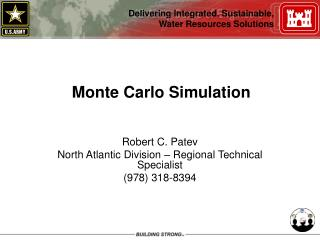# Monte Carlo Simulation - PowerPoint PPT PresentationDownload PresentationMonte Carlo Simulation

Monte Carlo Simulation
Download Presentation## Monte Carlo Simulation

- - - - - - - - - - - - - - - - - - - - - - - - - - - E N D - - - - - - - - - - - - - - - - - - - - - - - - - - -
##### Presentation Transcript

1. Monte Carlo Simulation Robert C. Patev North Atlantic Division – Regional Technical Specialist (978) 318-8394

2. “Monte Carlo” is the method (code name) for simulations relating to development of atomic bomb during WWII • Traditional – static not dynamic (not involve time), U(0,1) • Non-Traditional – multi-integral problems, dynamic (time) • Applied to wide variety of complex problems involving random behavior • Procedure that generates values of a random variable based on one or more probability distributions • Not simulation method per se – just a name!

3. Analytical solution • For many problems analytical solution difficult or may not exist • An approximate solution • Monte Carlo Simulation • Monte Carlo process • Frequently used in risk analysis to generate sample of realizations of the model • Not always necessary • Can enumerate all the end-points • Model collapsed into single period • Full enumeration may not be feasible • Simulation produces no new information about contributing variables • The analyst must provide the distributions for the contributing variables • Monte Carlo simulation is a numerical technique for estimating a distribution • Resulting statistics are reflection of sample

4. Simple Example: Determine the expected value and distribution of the sum of two die Each face of each die has equal probability = 1/6 Multiple ways of getting the same sum Analytical Solution: Enumerate all possible combinations EX. Pr(3) = Pr(1 and 2) + Pr(2 and 1) = 1/36+1/36 = 0.05556

5. Two Die Toss Distribution

6. Requirements for Monte Carlo Simulation (1)Model describing in quantitative terms the variable(s) of interest and the relationship among them (2)Estimate distributions of the contributing variables (3)Generate random numbers (4)Transform random numbers into useful values using a specific probability distribution (5)Criteria for determining sample size (6)Perform a statistical analysis on the random sample to determine

7. Results from Monte Carlo Simulation • Means often stabilize quickly--few hundred • Estimating probabilities of outcomes take MANY more • Defining tails of output distribution takes MANY MANY more iterations • If extreme events are important it make take MANY MANY MANY more • Convergence is key to a believable result from a simulation. Documentation is critical. • Depends on the degree of accuracy desired

8. Criteria for Determining Sample Size Number of iterations required increases with • increases in variance and skew • reductions in probability • the number of variables simulated • Many rules of thumb in literature….. • Really need to set and examine convergence criteria • Convergence • Sample mean • Sample variance • Extremes--maximum and minimum • Percentiles • Confidence intervals

9. Running Average

10. Stopping Rules • Some commercial Monte Carlo simulation software rely on convergence • User specified percentage change in sample statistics • Careful…..THIS MAY NOT BE TRUE CONVERGENCE OF THE LIMIT STATE! • Careful….CONVERGENCE ON TIME-DEPENDENT PROBLEMS NEEDS TO BE EXAMINED ON EACH TIME INCREMENT!